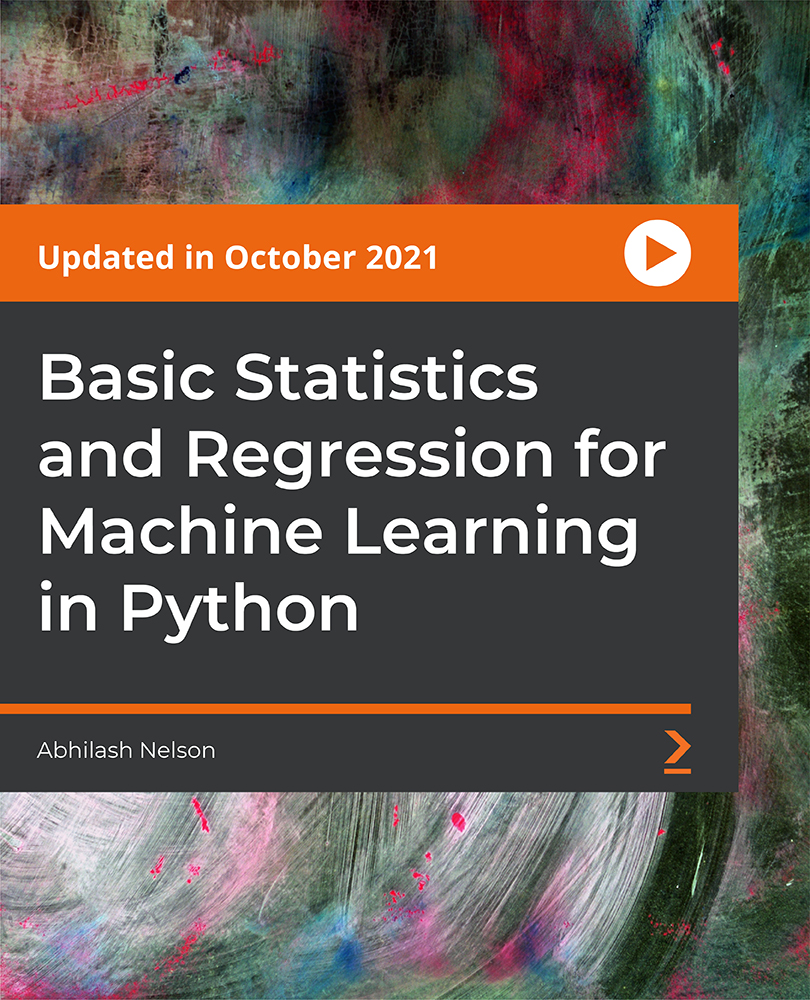# Basic Statistics and Regression for Machine Learning in Python

Get ready to learn the basics of machine learning and the mathematics of statistical regression, which powers almost all machine learning algorithms.

Language - English Published on 06/2022

10:16
Preview

#### Description

This course is for ML enthusiasts who want to understand basic statistics and regression for machine learning. The course starts with setting up the environment and understanding the basics of Python language and different libraries. Next, you’ll see the basics of machine learning and different types of data. After that, you’ll learn a statistics technique called Central Tendency Analysis.

Post this, you’ll focus on statistical techniques such as variance and standard deviation. Several techniques and mathematical concepts such as percentile, normal distribution, uniform distribution, finding z-score, linear regression, polynomial linear regression, and multiple regression with the help of manual calculation and Python functions are introduced as the course progresses.

The dataset will get more complex as you proceed ahead; you’ll use a CSV file to save the dataset. You’ll see the traditional and complex method of finding the coefficient of regression and then explore ways to solve it easily with some Python functions.

Finally, you’ll learn a technique called data normalization or standardization, which will improve the performance of the algorithms very much compared to a non-scaled dataset.

By the end of this course, you’ll gain a solid foundation in machine learning and statistical regression using Python.

All the code files and related files are available on the GitHub repository at https://github.com/PacktPublishing/Basic-Statistics-and-Regression-for-Machine-Learning-in-Python

Audience:

This course is for beginners and individuals who want to learn mathematics for machine learning. You need not have any prior experience or knowledge in coding; just be ready with your learning mindset at the highest level.

Individuals interested in learning what’s actually happening behind the scenes of Python functions and algorithms (at least in a shallow layman’s way) will be highly benefitted.

#### What Will I Get ?

• Set up the environment.
• Learn central tendency analysis.
• Learn statistical models and analysis.
• Learn regression models and analysis.
• Use NumPy, matplotlib, and scikit-learn libraries.
• Learn the data normalization or standardization technique.

#### Requirements

• Basic computer knowledge and an interest to learn mathematics for machine learning is the only prerequisite for this course.

#### Feedbacks (0)

• No Feedbacks Posted Yet..!This Course Includes :

5 hours

63 Lectures